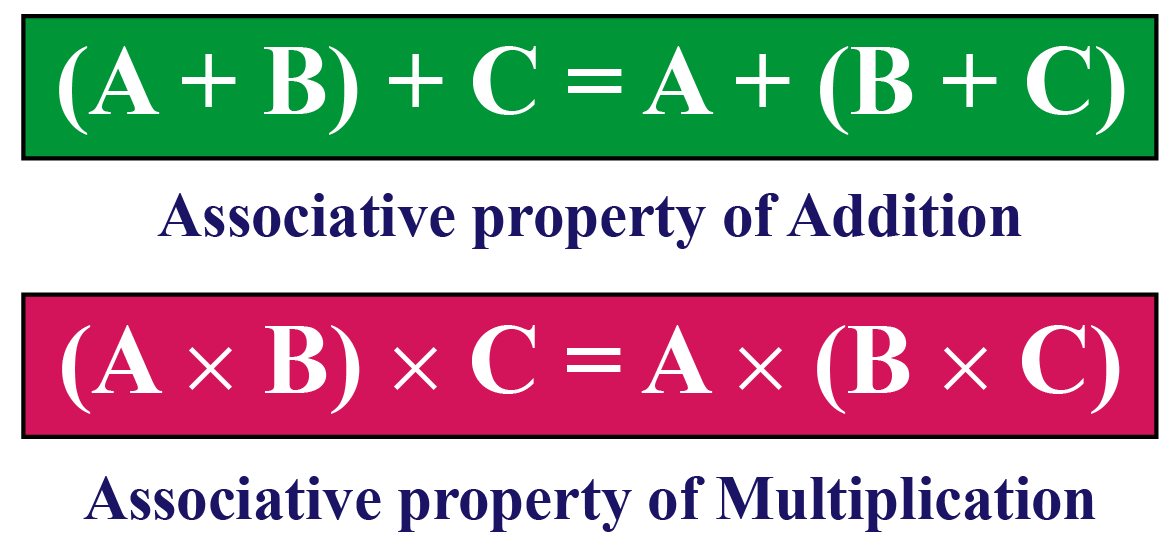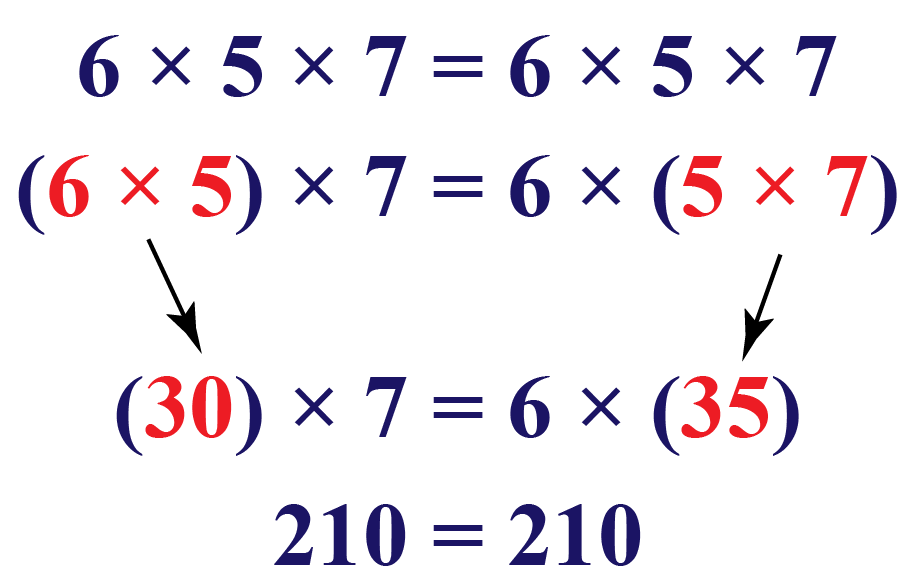# Associative Property of Multiplication

Associative Property of Multiplication
Go back to  'What are Numbers'

Don’t you think it’s easier to calculate $$5 \times (10 \times 10),$$ than $$10 \times (5 \times 10)?$$

In this mini-lesson, we will figure out why it is so and explore the world of the associative property of multiplication. The journey will start with the definition of the associative property and will lead us to interesting facts and exciting examples.You can check out the interactive calculator to know more about the lesson and try your hand at solving a few real-life practice questions at the end of the page.

What are we waiting for, let’s get started!

## What Is the Associative Property of Multiplication?

The Associative property tells us that we can add/multiply the numbers in an equation irrespective of the grouping of those numbers.

Grouping is mainly done using parenthesis.

Thus, associativity helps us in solving these equations regardless of the way they are put in parenthesis.

## Associative Property of Multiplication

We have already learned about Associative Property. So, let's take a step forward and understand the associative property of multiplication definition.

Before we get into the actual definition of the associative property of multiplication, let us take any general function (F) of multiplication as an example.

$$F = a \times b \times c$$

But, Associative Property of Multiplication tells us that

$$F = (a \times b) \times c = a \times (b \times c)$$

Take a look at how this property works from the example given below.Now try putting values of any 3 numbers here and see how Associative property for Multiplication works for other functions and numbers!

From the above example and simulation, we can say that the associative property of multiplication is defined as the property of multiplication where the product of three or more numbers remains the same regardless of how the numbers are grouped.

More Important Topics
Numbers
Algebra
Geometry
Measurement
Money
Data
Trigonometry
Calculus